# Uniform circular motion (MCU)

Uniform circular motion (MCU) occurs when an object executes a circular path with constant magnitude velocity.

Uniform circular motion (MCU) occurs when a piece of furniture moves along a circular path with a constant speed . . The importance of studying this movement is in understanding the operation of engines, systems composed of gears and pulleys, and can be applied even in the movement of natural and artificial satellites.

The MCU has acceleration!

The velocity vector of an object that performs uniform circular motion is tangent to the trajectory and has a constant magnitude (numerical value). When executing a curve, the velocity vector undergoes changes in direction and sense, therefore, there is centripetal acceleration (a CP ) .

Centripetal acceleration (the CP ) is responsible for changing the direction and direction of the velocity vector . In the figure above, it can be seen that the velocity vector, in addition to being tangent to the trajectory, has different directions and directions for different points of the curve. The centripetal acceleration (the CP ) is defined by the ratio between the square of the velocity (V) and the radius of the circular path (R).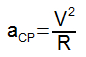Period and frequency

• Period (T) : is the time required for an object in circular motion to complete a turn;
• Frequency (f) : is the number of turns performed in a time interval. The units used for frequency are RPM (revolutions per minute) and Hz (revolutions per second). The International System of Units states that the unit of measurement for frequency must be the Hz.

Mathematically, the period is the inverse of the frequency, and the frequency is the inverse of the period.angular velocity

The angular velocity (ω) is the ratio of the angular displacement suffered by a mobile with respect to time.

Don’t stop now… There’s more after the publicity 😉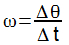According to the International System of Units , angular velocity is given in radians per second (rad/s).

Note that, in the displacement between points 1 and 2, there is an angular displacement of value θ. If every second of movement the same angle θ is “swept” by the line joining the moving object to the center of the trajectory, we can say that the object moves θ radians per second. This is the definition of angular velocity.

Angular velocity can be written in terms of frequency and period of motion . For this, we need to consider that, in a complete turn, the angular displacement is 360° (2 π rad) and that the time spent in a complete turn corresponds precisely to the period (T) of the movement: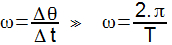Since the frequency is the inverse of the period, we can write: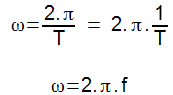Relationship between angular velocity and linear velocity

Knowing that linear velocity can be defined as the ratio between the distance traveled (Δs) and the time interval (Δt), we can determine a relationship between angular velocity and linear velocity for an object that performs circular motion.

For this, it is necessary to understand that, in a complete turn, the space covered corresponds to the length of the circumference (c = 2. π . R) and that the time taken for a complete turn is precisely the period (T) of the movement. Therefore, we can write: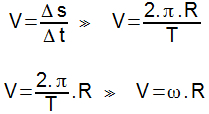The linear velocity (V) of a mobile that performs circular motion is given by the product of the angular velocity (ω) and the radius of the trajectory.

Check Also
Close